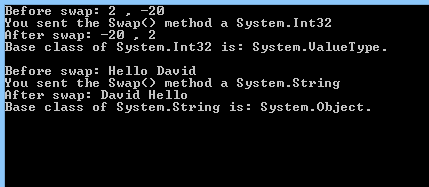# Programming with VB Using Visual Studio 2012

Objectives –

Delegates will learn to develop web applications using VB 4.0. After completing this course, delegates will be able to:

• Use Visual Studio 2012 effectively
• Programming with VB using Visual Studio 2012

Audience

This course has been designed primarily for programmers new to the .Net development platform. Delegates experience solely in Windows application development or earlier versions of ASP.Net will also find the content beneficial.

Prerequisites

Before attending this workshop, students must:

• Be able to manage a solution environment using the Visual Studio 2012 IDE and tools
• Be able to program an application using a .NET Framework 4.0 compliant language

Contents

Lab 1 – VB Language Fundamentals
In this lab we will start to work with VB. We will do this by creating Console Applications in Visual Studio 2012. Then write statements that prompt and greet the user.

1. Open Visual Studio 2012 and create a new VB Console Application:
• Select the File menu, then select New Project OR (CTRL+Shift+N).
• On the left menu, Expand Templates then expand Visual VB and select Windows
• Choose Console Application.
• Name the project and the solution: VBLanguageFundamentals
• Browse to the place on your computer where you wish Visual Studio

to create the directory for your solution (keeping the tick box, “Create Directory For Solution”, selected ) or leave the default location.

• Click Ok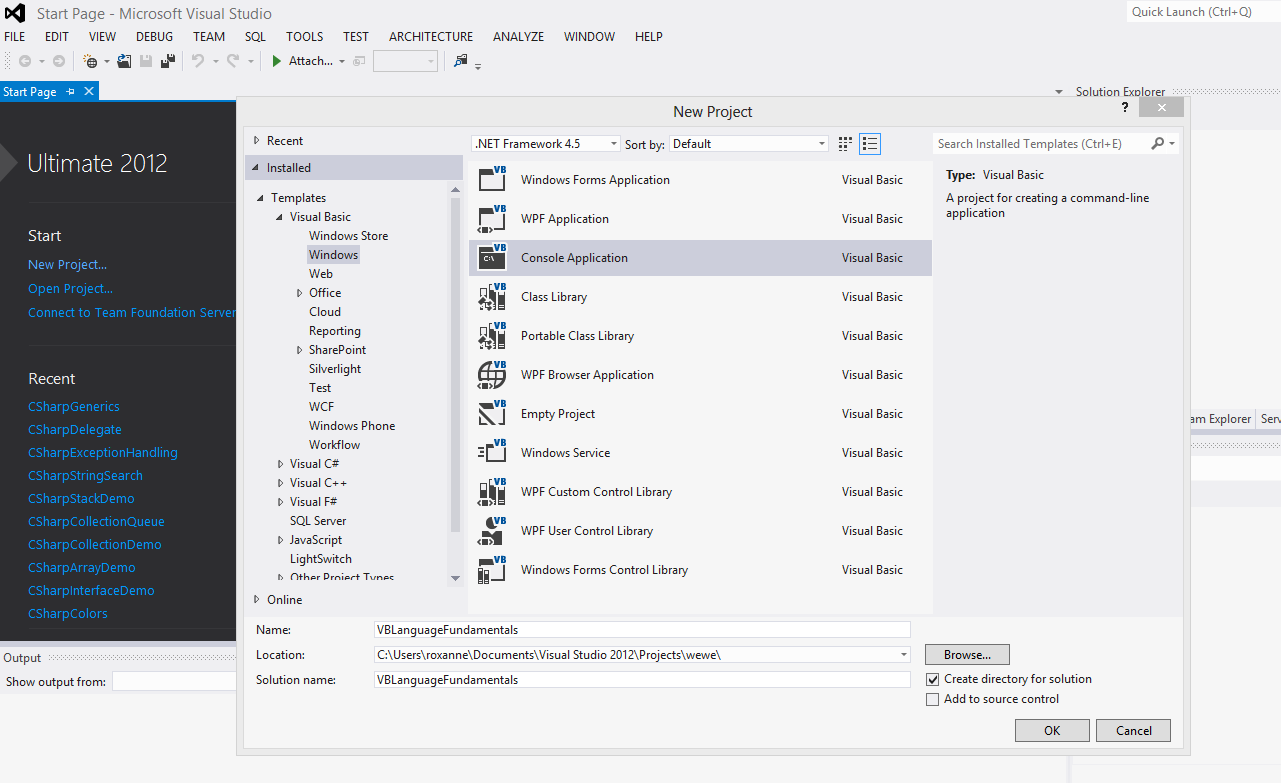2. By default the class Program.cs is opened. Declare a variable, ‘myName’, of type String. Find the Main method and insert the following line:
Dim myName As String
3. Write a statement that prompts the user for his/her name
4. Write another statement that reads the user’s input from the keyboard and assigns it to the ‘myName’ string.
5. Add one more statement that prints “Hello myName” to the screen (where myName is the name the user has typed).
6. When completed, the Main method should contain the following:
Sub Main()
‘This is a comment:

‘Declare the variable “myName” of type string
Dim myName As String

‘prompt the user to enter his/her name

‘The name entered will be assigned to the variable “myName”

‘Displaye “Hello myName”
Console.WriteLine(“Hello {0}”, myName)

‘vbCrLf: Display a new line
Console.WriteLine(vbCrLf + “Please Press any Key to Exit”)
‘Prevents the program from exiting immediately when the code has
‘finished executing
End Sub

7. Test the application and save your work; You can run the application by clicking on the Start button with the green arrow
8.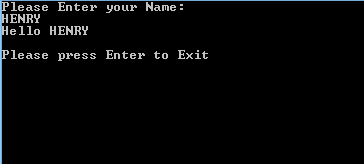9. View code file

Lab 2 – Branching

Create Console Applications that uses conditional and branching statements.

Complete three simple programming tasks in VB. For each solution create a new Console Application.

Exercise 1
Sum of two positive integers

1. Open Visual Studio and create a new VB Console Application. (Same as Lab 1 > first point). The Solution name and the project name is VBSumOf2Numbers
2. Tip:It’s a good practice to add comments to your code. As you notice, in the code below we added some comments that makes the code more comprehensible. In order to add a 1 line comment write a single quote before your comment; anything after ‘ is ignored by the compiler.
3. Write statements to input two positive integers and display the sum.
‘Declaring variables
Dim valueOne As Integer
Dim valueTwo As Integer
Dim valueThree As Integer
‘Try…Catch: is used to catch any unexpected behavior
Try
‘Prompt the user to enter the first operand
System.Console.WriteLine(“Please Enter A Whole Number: “)
‘Prompt the user to enter the second operand
System.Console.WriteLine(“Please Enter Another Whole Number: “)
‘Calculate the sum of the 2 variables
valueThree = valueOne + valueTwo
‘Print if sum > 0
If (valueThree > 0) Then
System.Console.WriteLine(“The Sum of ValueOne: {0}
and ValueTwo: {1} is Equal to: {2}”, valueOne, valueTwo,
valueThree)
Else ‘Else if the sum is NOT greater than 0
System.Console.WriteLine(“Both Values were Zero!”)
End If
Catch ex As System.FormatException
Console.WriteLine(“You didn’t enter a Whole Number!”)
End Try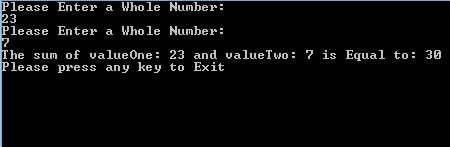5. View code file

Exercise 2
Largest Number Among 3 Numbers

1. Open Visual Studio and create a new VB Console Application. (Same as Lab 1 > first point). The Solution name and the project name is VBGreatestNumber
2. Write statements to input three numbers and display the largest using if statements. Aim to use the minimum number of statements.
3. Only if the condition of the if statement is true the block inside the it is executed
‘Compare the numbers to find out which is the highest number
intMax = intFirst
If (intSecond > intMax) Then
intMax = intSecond
End If
If (intThird > intMax) Then
intMax = intThird
End If
‘Show the largest
Console.WriteLine(“The largest number entered is: {0}”, intMax)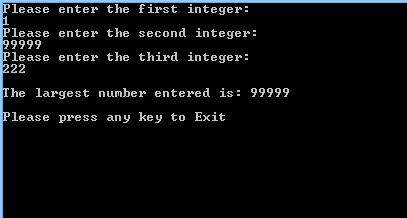5. View code file.

Exercise 3
Switch – Get the capital

1. Open Visual Studio and create a new VB Console Application. (Same as Lab 1 > first point). The Solution name and the project name is VBSwitch
2. Write a select statement to input a country and display its capital.
‘Declare a variable “strMyCountry” of type string
Dim strMyCountry As String
‘Prompt the user to enter a country
‘Use select to compare the myCountry string to the choices
Select Case (strMyCountry)
Case “England”
‘This block is executed only
‘if the country entered by the user is “England”
‘(case sensitive)
Console.WriteLine(“Your Capital is London.” + vbCrLf)
Case “France”
Console.WriteLine(“Your Capital is Paris.” + vbCrLf)
Case “Germany”
Console.WriteLine(“Your Capital is Munich.” + vbCrLf)
Case Else
‘This block is executed only if the country entered is different
‘than above countries
Console.WriteLine(“The Capital is Unknown.” + vbCrLf)
End Select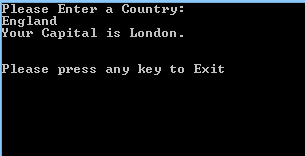4. View code file

Exercise 4
For loop – Display 10, 20, 30 …100

1. Open Visual Studio and create a new VB Console Application. (Same as Lab 1 > first point). The Solution name and the project name is VBForLoopCounter1
2. The for loop block in the following code will be executed 100 times.
3. Write statements that display the sequence 10, 20, 30, 40 … 100. Use a for loop.
‘Initialize counter
Dim counter As Integer = 0
‘Use for loop to generate values from 1 to 100 (Inclusive)
For counter = 1 To 100
‘print out counter when it can be divided by 10
If (counter Mod 10 = 0) Then
Console.WriteLine(“counter: {0} “, counter)
End If
Next counter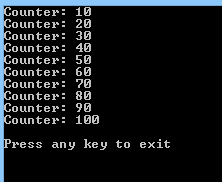5. View code file.

Exercise 5
For Loop – Sequence 1 to 100

1. Open Visual Studio and create a new VB Console Application. (Same as Lab 1 > first point). The Solution name and the project name is VBForLoopCounter2
2. Write statements that to display the sequence 1, 2, 3, … 100, as 10 rows of 10 numbers. Use a for loop.
‘declaration
Dim counter As Integer
Dim strNumberRow As String
counter = 0
strNumberRow = “”

‘Use for loop to count from 1 to 100
For counter = 1 To 100
If (counter < 10) Then
strNumberRow += ” “
End If
‘If the number can be divided by 10 display it with a new line
If (counter Mod 10 = 0) Then
strNumberRow += Convert.ToString(counter) + vbCrLf
Else ‘else display it with a space
strNumberRow += Convert.ToString(counter) + ” “
End If
Next counter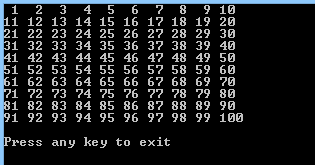4. View code file

Lab 3 – Operators

Create a Console Application that uses arithmetic and logical operators. Complete an arithmetic and a logical programming task.

Exercise 1
Multiply by 12

1. Open Visual Studio and create a new VB Console Application. (Same as Lab 1 > first point). The Solution name and the project name is VBMultiplication
2. Write statements using the multiplication operator to display the twelve-times table. Enter a number from 1 to 12, then display the number multiplied by 12.
‘Declare Variables of type integer
Dim valueOne As Integer
Dim valueTwo As Integer
‘Const variable can not be modified
Const multiplyer As Integer = 12
Try
Console.WriteLine(“Please enter a number between 1 and 12”)
‘Check if number is between 1 and 12
If (valueOne > 0 And valueOne < 13) Then
valueTwo = valueOne * multiplyer
Console.WriteLine(“{0} x {1} = {2}”, valueOne, multiplyer,
valueTwo)
Else
Console.WriteLine(“You didn’t enter a number between 1 and 12”)
End If
Catch ex As System.FormatException
Console.WriteLine(“You didn’t enter a Whole Number!”)
End Try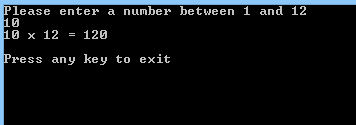4. View code file.

Exercise 2
Multiplication Sign

1. Open Visual Studio and create a new VB Console Application. (Same as Lab 1 > first point). The Solution name and the project name is VBPositiveOrNegative
2. Write statements to input two numbers and use logical operators to output if the result of multiplying them will be positive or negative. For example, a negative number multiplied by a negative number, gives a positive result.
‘Declare 3 integer
Dim valueOne As Integer
Dim valueTwo As Integer
Dim valueThree As Integer
Try
‘Prompt the user to enter 3 numbers
Console.WriteLine(“Please enter a number greater or less than 0”)
Console.WriteLine(“Please enter another number greater or
&nbspless than 0″)
valueThree = valueOne * valueTwo
If (valueOne > 0 And valueTwo > 0) Then
ElseIf (valueOne < 0 And valueTwo < 0) Then
ElseIf (valueOne < 0 Or valueTwo < 0) Then
Else
Console.WriteLine(“Both numbers are not greater or less than 0”)
End If
Catch ex As System.FormatException
Console.WriteLine(“You didn’t enter a Whole Number!”)
End Try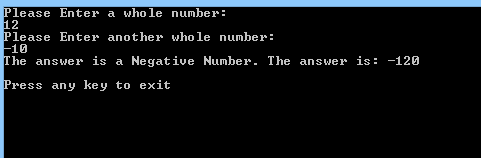4. View code file.

Lab 4 – Debugging

Examine and debug your VB code by using Visual Studio’s debugging features. These in include pausing code at a breakpoint, stepping through statements and using the debug windows.

For all remaining lab exercises throughout this course, examine and debug your code by:

1. Setting breakpoints in lines of code by clicking in the margin. When run the code will pause at the first beakpoint.
2. Stepping through lines of code when a breakpoint is hit by pressing F11. The code will execute line by line. Hover the mouse pointer over a variable to show its current value.
3. Using these debug windows to understand and fix code:
• Watch
• Locals
• Immediate
• Call Stack
4. Debug windows are available only when the code is paused in break mode. Use the Debug>Windows command to show them.

Lab 5 – Structs

Create a Structure to hold colour values. Use the Structure in code.

1. Open Visual Studio and create a new VB Console Application. (Same as Lab 1 > first point). The Solution name and the project name is VBColors
2. Define a structure called Colour, with a constructor and a ToString() method.
3. Add three integer properties to represent the red, green and blue component of the colour.
‘declare a struct named Colour
Public Structure Colour
‘Properties
Public Property Red As Integer
Public Property Green As Integer
Public Property Blue As Integer
‘Constructor
Public Sub New(ByVal rVal As Integer, ByVal gVal As Integer, ByVal bVal As Integer)
Red = rVal
Green = gVal
Blue = bVal
End Sub
‘Display the Struct as a String
Public Overrides Function ToString() As String
Return String.Format(“{0}, {1}, {2}”, Red, Green, Blue)
End Function
End Structure
4. Test the struct.
Public Class Tester
Public Sub Run()
‘Create an instance of the struct
Dim colour1 As Colour = New Colour(100, 50, 250)
‘Display the values
Console.WriteLine(“Colour1 Initialized using the non-default
constructor: “)
Console.WriteLine(“colour1 colour: {0}”, colour1)
Console.WriteLine()
‘Invoke the default constructor
Dim colour2 As Colour = New Colour()
Console.WriteLine(“Colour2 Initialized using the default
constructor: “)
Console.WriteLine(“colour2 colour: {0}”, colour2)
Console.WriteLine()
‘Pass the struct object to a method
MyMethod(colour1)
‘Redisplay the values in the struct
Console.WriteLine(“Colour1 after calling the method
‘MyMethod’: “)
Console.WriteLine(“colour1 colour: {0}”, colour1)
Console.WriteLine()
End Sub
‘Method takes a struct as a parameter
Public Sub MyMethod(ByVal col As Colour)
‘Modify the values through the properties
col.Red = 200
col.Green = 100
col.Blue = 50
Console.WriteLine(“colour values from inside the ‘MyMethod’
method: {0}”, col)
Console.WriteLine()
End Sub
End Class

Sub Main()
Dim t As Tester = New Tester()
t.Run()
End Sub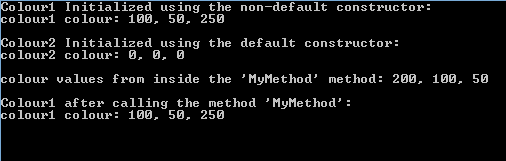6. View code file.

Lab 6 – Interfaces
Create an Interface and a Class to hold client details. Instantiate an object from the class in code.

1. Open Visual Studio and create a new VB Console Application. (Same as Lab 1 > first point). The Solution name and the project name is VBInterfaceDemo
2. Define an interface called IClient.
Interface IClient
Sub Order(ByVal orderNum As Integer)
Property Name() As String
End Interface
3. Create a second class called Customer that implements all the interface’s members.
‘Create a Customer class that implements the IClient interface
Public Class Customer Implements IClient
‘Implement the properties
Public Property Name As String Implements IClient.Name
Public Sub New(ByVal s As String)
Console.WriteLine(“Creating a New Customer ID: {0}”, s)
End Sub
‘Implement the Order method
Public Sub Order(ByVal newOrder As Integer) Implements IClient.Order
Console.WriteLine(“Implementing the Order Method for IClient.
The Order Number is: {0}”, newOrder)
End Sub
End Class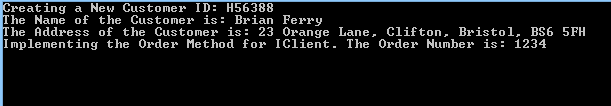5. View code file.

Lab 7 – Arrays

Create a Console Application that uses a two dimensional array to hold data. Populate the array with integers using a loop.

1. Open Visual Studio and create a new VB Console Application. (Same as Lab 1 > first point). The Solution name and the project name is VBArrayDemo
2. Create a two dimensional array of integers.
‘Declare the multi-dimensional array
Dim intArray(,) As Integer
‘Initialise the mutli-dimensional array with 10 rows and 10 columns
intArray = New Integer(9, 9) {}
3. Use looping statements to populate the array.
‘Loop over all the elements
For j = 0 To intArray.GetLength(1) – 1
For k = 0 To intArray.GetLength(1) – 1
‘ the intValue variable will count from 1 to a 100
intValue += 1
intArray(j, k) = intValue
If (intValue < 10) Then
numberRow += intArray(j, k).ToString + ” “
Else
numberRow += intArray(j, k).ToString + ” “
End If
Next k
‘Print out the row of numbers
Console.WriteLine(numberRow)
numberRow = ” “
Next j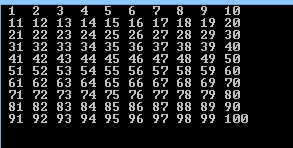5. View code file.

Lab 8 – Collection Interfaces and Types

Populate an ArrayList, Stack and Queue collections. Then display the values.

Exercise 1
Creating an ArrayList

1. Open Visual Studio and create a new VB Console Application. (Same as Lab 1 > first point). The Solution name and the project name is VBCollectionDemo
2. Create an ArrayList of integers. Use looping statements to populate this with multiples of 10. Print each member of the list. Experiment with the ArrayLists methods, including the Sort(), Reverse() and Clear() methods.
‘Initialize an empty Array list
Dim intArray As ArrayList = New ArrayList()
‘Declare an integer
Dim i As Integer
‘Populate the arraylist
‘Add 5 integers: 10, 20, 30, 40 and 50
For i = 0 To 4
Next i
‘Print each member of the ArrayList
Console.WriteLine(“Displaying the contents of the array list “)
For Each j As Integer In intArray
Console.Write(“{0} “, j.ToString())
Next j
‘Reverse
intArray.Reverse()
Console.WriteLine(vbCrLf + vbCrLf + “Now the list is reversed “)
For Each j As Integer In intArray
Console.Write(“{0} “, j.ToString())
Next j
‘Sort
intArray.Sort()
Console.WriteLine(vbCrLf + vbCrLf + “Now the order of the list has been sorted “)
For Each j As Integer In intArray
Console.Write(“{0} “, j.ToString())
Next j
‘Clear
intArray.Clear()
Console.WriteLine(vbCrLf + vbCrLf + “Now the list has been cleared “)
If (intArray.Count() = 0) Then
Console.WriteLine(“There array list is empty”)
End If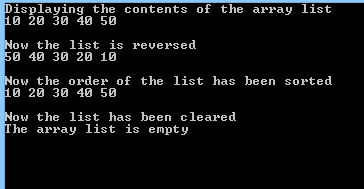4. View code file.

Exercise 2
Creating a Queue

1. Open Visual Studio and create a new VB Console Application. (Same as Lab 1 > first point). The Solution name and the project name is VBCollectionQueue
2. Create a Queue of integers. Use looping statements to populate this. Experiment with the Queues methods, including the Dequeue(), Enqueue () and Peek() methods.
Dim intQueue As Queue = New Queue()
Dim i As Integer
‘Populate the Queue
For i = 0 To 4
intQueue.Enqueue((i + 1) * 10)
Next i
‘Print each member
Console.WriteLine(“Here are the items in the Queue: “)
For Each j As Integer In intQueue
Console.Write(“{0} “, j.ToString())
Next j
‘Displays the first member
Console.Write(vbCrLf + “The value of the first member is: {0}”
+ vbCrLf, intQueue.Peek().ToString())
‘Removes the first item from the Queue and displays the remaining items
intQueue.Dequeue()
Console.Write(“The first item has now been removed.”
+ vbCrLf + “Now the remaining members are: “)
‘Print each member of the Queue
For Each j As Integer In intQueue
Console.Write(“{0} “, j.ToString())
Next j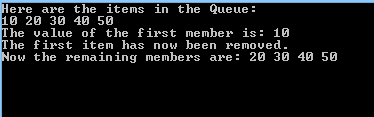4. View code file

Exercise 3
Creating a Stack

1. Open Visual Studio and create a new VB Console Application. (Same as Lab 1 > first point). The Solution name and the project name is VBStackDemo
2. Create a Stack of integers. Use looping statements to populate this. Experiment with the Stacks methods, including the Pop(), Push () and Peek() methods.
‘Initialize a new stack
Dim intStack As Stack = New Stack()
Dim i As Integer
‘Populate the Stack
For i = 0 To 5
intStack.Push((i + 1) * 10)
Next i
‘Print each member of the Stack
Console.WriteLine(“Here are the items in the Stack: “)
For Each j As Integer In intStack
Console.Write(“{0} “, j.ToString())
Next j
‘Display the topmost member of the Stack
Console.Write(vbCrLf + “The value of the topmost member is: {0} “
+ vbCrLf, intStack.Peek().ToString())
‘Removes and display
intStack.Pop()
Console.Write(“The topmost item has now been removed.”
+ vbCrLf + “Now the remaining members are: “)
‘Print each member of the Stack
For Each j As Integer In intStack
Console.Write(“{0} “, j.ToString())
Next j
3. Run the application: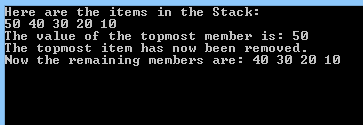4. View code file.

Lab 9 – Strings
Create a Console Application with strings to hold textual data. Then extract a substring from a string. Also split a string into parts. Finally loop through an array of strings.

1. Open Visual Studio and create a new VB Console Application. (Same as Lab 1 > first point). The Solution name and the project name is VBStringSearch
2. Initialize a string with value = e.g. http://www.bbc.co.uk .
3. Experimenting with extracting substrings, e.g. “bbc”.
‘Create some strings to work with
Dim s1 As String = “http://www.bbc.co.uk”
Dim s2 As String
Dim s3 As String
Dim index As String
‘Substring method takes 2 parameters:
‘from index (Inclusicve) and To index (Exclusive)
‘Search for first substring
s2 = s1.Substring(0, 4)
Console.WriteLine(s2)
‘Search for next substring
index = s1.LastIndexOf(“/”)
s2 = s1.Substring(index + 1, 3)
Console.WriteLine(s2)
‘Search for next substring
index = s1.IndexOf(“.”)
s2 = s1.Substring(index + 1)
s3 = s2.Substring(0, 3)
Console.WriteLine(s3)
‘Search for next substring
index = s2.IndexOf(“.”)
s3 = s2.Substring(index + 1, 2)
Console.WriteLine(s3)
‘Search for last substring
index = s1.LastIndexOf(“.”)
s3 = s1.Substring(index + 1, 2)
Console.WriteLine(s3)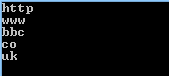5. View code file.

Lab 10 – Throwing and Catching Exceptions

Add exception handling to an application. When an exception (error) occurs the code branches to an exception handler. The handler can then take action to resolve the problem.

1. Open Visual Studio and create a new VB Console Application. (Same as Lab 1 > first point). The Solution name and the project name is VBExceptionHandling
2. Create a console application to enter two integers, then divide the numbers.
Try
‘Declare variable
Dim a As Double
Dim b As Double
Console.WriteLine(“Dividing {0} by {1}…”, a, b)
Console.WriteLine(“{0} / {1} = {2}”, a, b, DoDivide(a, b))
‘Most derived exception type first
Catch e As System.DivideByZeroException
‘This block is executed ONLY IF the user enters 0 as a divider
Console.WriteLine(vbCrLf + “DivideByZeroException! Msg: {0}”, e.Message)
Catch e As System.ArithmeticException
‘This block is executed ONLY IF the user enters 0 as the first number
Console.WriteLine(vbCrLf + “ArithmeticException! Msg: {0}”, e.Message)
‘Generic exception type last
Catch
Console.WriteLine(“Unknown exception caught”)
Finally
Console.WriteLine(“Close file here.”)
End Try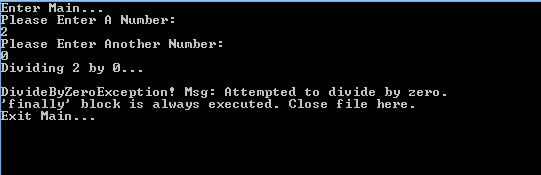4. View code file.

Lab 11 – Delegates

A delegate is a pointer to a method. After declaring a delegate, it can hold any method, providing the number and type of parameters match the delegate’s declaration.

1. Open Visual Studio and create a new VB Console Application. (Same as Lab 1 > first point). The Solution name and the project name is VBDelegate
2. Create a class Pair and include a delegate called WhichisFirst, a constructor, and methods called Sort() and ReverseSort().
Public Class Pair
‘Private array to hold the two objects
Private thePair(2) As Object
‘The delegate declaration
Public Delegate Function WhichIsFirst(ByVal obj1 As Object, ByVal obj2 As Object) As comparison
‘Passed in constructor takes two objects
Public Sub New(ByVal firstObject As Object, ByVal secondObject As Object)
thePair(0) = firstObject
thePair(1) = secondObject
End Sub
‘Sort method
Public Sub Sort(ByVal theDelegatedFunc As WhichIsFirst)
If (theDelegatedFunc(thePair(0), thePair(1)) = comparison.theSecondComesFirst) Then
Dim temp As Object = thePair(0)
thePair(0) = thePair(1)
thePair(1) = temp
End If
End Sub
‘Reverse Sort method
Public Sub ReverseSort(ByVal theDelegatedFunc As WhichIsFirst)
If (theDelegatedFunc(thePair(0), thePair(1)) = comparison.theFirstComesFirst) Then
Dim temp As Object = thePair(0)
thePair(0) = thePair(1)
thePair(1) = temp
End If
End Sub
‘Oberride ToString method
Public Overrides Function ToString() As String
Return thePair(0).ToString() + “, ” + thePair(1).ToString()
End Function
End Class
3. The sort methods will take as a parameters an instance of the WhichisFirst delegate.
Dim Milo As Dog = New Dog(65)
Dim Fred As Dog = New Dog(12)
Dim dogPair As Pair = New Pair(Milo, Fred)
Console.WriteLine(“dogPair” + vbTab + vbTab + vbTab + vbTab + “: {0}”, dogPair.ToString())
‘Instantiate the delegate
Dim theDogDelegate As Pair.WhichIsFirst = New Pair.WhichIsFirst(AddressOf Dog.WhichDogComesFirst)
‘Sort using the delegate
dogPair.Sort(theDogDelegate)
Console.WriteLine(“After Sort dogPair” + vbTab + vbTab + “: {0}”, dogPair.ToString())
dogPair.ReverseSort(theDogDelegate)
Console.WriteLine(“After ReverseSort dogPair” + vbTab + “: {0}”, dogPair.ToString())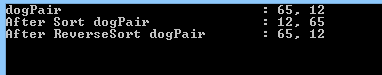5. View code file.

Lab 12 – Generics

Generics allow the type of a parameter to be changed at runtime. This allows the creation of very generalised classes and methods, that can work with any type of data. There are lots of useful predefined generic collections in the .Net framework.

1. Open Visual Studio and create a new VB Console Application. (Same as Lab 1 > first point). The Solution name and the project name is CSharpGenerics
2. Create a class that is generic.
3. Add generic methods to the class.
Public Class Generics(Of T) ‘Generic class
Public Sub Swap(Of K)(ByRef lhs As K, ByRef rhs As K)
‘Generic method
Console.WriteLine(“You sent the Swap() method a {0}”, GetType(K))
Dim temp As K ‘Local generic data type
temp = lhs
lhs = rhs
rhs = temp
End Sub
‘Displays the data type and its base type
Public Sub DisplayBaseClass(Of K)()
Dim i As Type
i = GetType(K)
Console.WriteLine(“Base class of {0} is: {1}.”, i, i.BaseType)
End Sub
End Class
4. Test the generic class
Dim g As Generics(Of Integer) = New Generics(Of Integer)
Dim a As Integer = 2
Dim b As Integer = -20
Console.WriteLine(“Before swap: {0} , {1}”, a, b)
g.Swap(a, b)
Console.WriteLine(“After swap: {0} , {1}”, a, b)
g.DisplayBaseClass(Of Integer)()
Dim gb As Generics(Of String) = New Generics(Of String)()
Dim strA As String = “Hello”, strB = “David”
Console.WriteLine(vbCrLf + “Before swap: {0} {1}”, strA, strB)
g.Swap(strA, strB)
Console.WriteLine(“After swap: {0} {1}”, strA, strB)
g.DisplayBaseClass(Of String)()